# 37 Results

View
Selected filters:
• Perimeter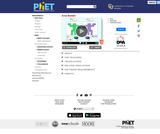Conditions of Use:
No Strings Attached
Rating

Create your own shapes using colorful blocks and explore the relationship between perimeter and area. Compare the area and perimeter of two shapes side-by-side. Challenge yourself in the game screen to build shapes or find the area of funky figures. Try to collect lots of stars!

Subject:
Mathematics
Material Type:
Simulation
Provider:
Provider Set:
PhET Interactive Simulations
Author:
Amanda McGarry
Ariel Paul
Bryce Gruneich
John Blanco
Kathy Perkins
01/08/2018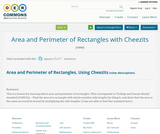Conditions of Use:
No Strings Attached
Rating

This is a lesson for learning about area and perimeter of rectangles.

This corresponds to "College and Career Ready" standard (3.MD.7a) -- Find the area of a rectangle with whole-number side lengths by tiling it, and show that the area is the same as would be found by multiplying the side lengths.
(I was not able to find that standard here.)

Subject:
Mathematics
Material Type:
Lesson Plan
Author:
Susan Jones
01/28/2017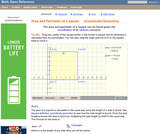Conditions of Use:
Rating

An interactive applet and associated web page showing how to find the area and perimeter of a square from the coordinates of its vertices. The square can be either parallel to the axes or rotated. The grid and coordinates can be turned on and off. The area and perimeter calculation can be turned off to permit class exercises and then turned back on the verify the answers. The applet can be printed as it appears on the screen to make handouts. The web page has a full description of the method for determining area and perimeter, a worked example and has links to other pages relating to coordinate geometry. Applet can be enlarged to full screen size for use with a classroom projector. This resource is a component of the Math Open Reference Interactive Geometry textbook project at http://www.mathopenref.com.

Subject:
Geometry
Material Type:
Simulation
Provider:
Math Open Reference
Author:
John Page
02/16/2011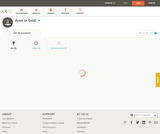Conditions of Use:
Remix and Share
Rating

This short video and interactive assessment activity is designed to teach third graders about area in grid.

Subject:
Mathematics
Measurement and Data
Material Type:
Assessment
Interactive
Lecture
Provider:
CK-12 Foundation
Provider Set:
CK-12 Elementary Math
03/04/2015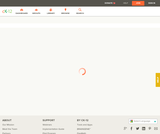Conditions of Use:
Remix and Share
Rating

This short video and interactive assessment activity is designed to teach third graders an overview of area of squares and rectangles - word problems.

Subject:
Mathematics
Measurement and Data
Material Type:
Assessment
Interactive
Lecture
Provider:
CK-12 Foundation
Provider Set:
CK-12 Elementary Math
03/04/2015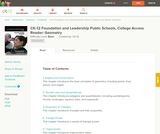Conditions of Use:
Remix and Share
Rating

This book is a "flexed" version of CK-12's Basic Geometry that aligns with College Access Geometry and contains embedded literacy supports. It covers the essentials of geometry for the high school student.

Subject:
Geometry
Material Type:
Textbook
Provider:
CK-12 Foundation
Provider Set:
CK-12 FlexBook
Author:
Fauteux, Michael
Zapata, Rosamaria
05/18/2011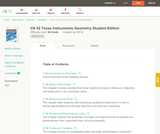Conditions of Use:
Remix and Share
Rating

CK-12 Foundation's Geometry FlexBook is a clear presentation of the essentials of geometry for the high school student. Topics include: Proof, Congruent Triangles, Quadrilaterals, Similarity, Perimeter & Area, Volume, and Transformations.

Subject:
Geometry
Material Type:
Textbook
Provider:
CK-12 Foundation
Provider Set:
CK-12 FlexBook
10/06/2009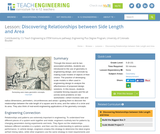Conditions of Use:
Rating

Through this lesson and its two associated activities, students are introduced to the use of geometry in engineering design, and conclude by making scale models of objects of their choice. The practice of developing scale models is often used in engineering design to analyze the effectiveness of proposed design solutions. In this lesson, students complete fencing (square) and fire pit (circle) word problems on two worksheets—which involves side and radius dimensions, perimeters, circumferences and areas—guiding them to discover the relationships between the side length of a square and its area, and the radius of a circle and its area. They also think of real-world engineering applications of the geometry concepts.

Subject:
Mathematics
Geometry
Measurement and Data
Material Type:
Lesson
Provider:
TeachEngineering
Author:
Aaron Lamplugh
Malinda Zarske
Nathan Coyle
Russell Anderson
Ryan Sullivan
02/07/2017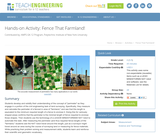Conditions of Use:
Rating

Students develop and solidify their understanding of the concept of "perimeter" as they engage in a portion of the civil engineering task of land surveying. Specifically, they measure and calculate the perimeter of a fenced in area of "farmland," and see that this length is equivalent to the minimum required length of a fence to enclose it. Doing this for variously shaped areas confirms that the perimeter is the minimal length of fence required to enclose those shapes. Then students use the technology of a LEGO MINDSTORMS(TM) NXT robot to automate this task. After measuring the perimeter (and thus required fence length) of the "farmland," students see the NXT robot travel around this length, just as a surveyor might travel around an area during the course of surveying land or measuring for fence materials. While practicing their problem solving and measurement skills, students learn and reinforce their scientific and geometric vocabulary.

Subject:
Engineering
Material Type:
Activity/Lab
Provider:
TeachEngineering
Provider Set:
TeachEngineering
Author:
Ursula Koniges
09/18/2014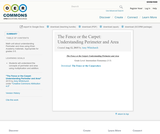Conditions of Use:
No Strings Attached
Rating

Subject:
Mathematics
Material Type:
Assessment
Homework/Assignment
Lesson Plan
Author:
Amy Whitchurch
01/28/2016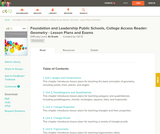Conditions of Use:
Remix and Share
Rating

A structured geometry program teacher edition of daily lesson plans and teacher supports to accompany the College Access Reader: Geometry student edition.

Subject:
Geometry
Material Type:
Textbook
Provider:
CK-12 Foundation
Provider Set:
CK-12 FlexBook
Author:
Fauteux, Michael
Zapata, Rosamaria
05/18/2011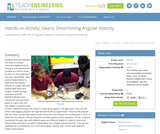Conditions of Use:
Rating

Students work as engineers and learn to conduct controlled experiments by changing one experimental variable at a time to study its effect on the experiment outcome. Specifically, they conduct experiments to determine the angular velocity for a gear train with varying gear ratios and lengths. Student groups assemble LEGO MINDSTORMS(TM) NXT robots with variously sized gears in a gear train and then design programs using the NXT software to cause the motor to rotate all the gears in the gear train. They use the LEGO data logging program and light sensors to set up experiments. They run the program with the motor and the light sensor at the same time and analyze the resulting plot in order to determine the angular velocity using the provided physics-based equations. Finally, students manipulate the gear train with different gears and different lengths in order to analyze all these factors and figure out which manipulation has a higher angular velocity. They use the equations for circumference of a circle and angular velocity; and convert units between radians and degrees.

Subject:
Engineering
Material Type:
Activity/Lab
Provider:
TeachEngineering
Provider Set:
TeachEngineering
Author:
James Cox
Jasmin Mejias
Jennifer S. Haghpanah
Leonarda Huertas
Mihai Pruna
09/18/2014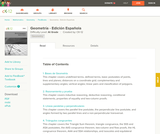Conditions of Use:
Remix and Share
Rating

CK-12 Foundation's Geometry FlexBook is a clear presentation of the essentials of geometry for the high school student. Topics include: Proof, Congruent Triangles, Quadrilaterals, Similarity, Perimeter & Area, Volume, and Transformations.

Subject:
Geometry
Material Type:
Textbook
Provider:
CK-12 Foundation
Provider Set:
CK-12 FlexBook
11/23/2010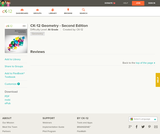Conditions of Use:
Remix and Share
Rating

CK-12's Geometry - Second Edition is a clear presentation of the essentials of geometry for the high school student. Topics include: Proofs, Triangles, Quadrilaterals, Similarity, Perimeter & Area, Volume, and Transformations.

Subject:
Geometry
Material Type:
Textbook
Provider:
CK-12 Foundation
Provider Set:
CK-12 FlexBook
Author:
Dirga, Kathryn
Jordan, Lori
11/16/2010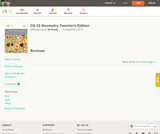Conditions of Use:
Remix and Share
Rating

CK-12 Geometry Teacher's Edition covers tips, common errors, enrichment, differentiated instruction and problem solving for teaching CK-12 Geometry Student Edition. The solution and assessment guides are available upon request.

Subject:
Geometry
Material Type:
Textbook
Provider:
CK-12 Foundation
Provider Set:
CK-12 FlexBook
Author:
Kershaw, Jem
Kramer, Melissa
Zwack, Teresa
06/25/2011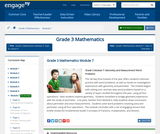Conditions of Use:
Remix and Share
Rating

This 40-day final module of the year offers students intensive practice with word problems, as well as hands-on investigation experiences with geometry and perimeter.  The module begins with solving one- and two-step word problems based on a variety of topics studied throughout the year, using all four operations.  Next students explore geometry.  Students tessellate to bridge geometry experience with the study of perimeter.  Line plots, familiar from Module 6, help students draw conclusions about perimeter and area measurements.  Students solve word problems involving area and perimeter using all four operations.  The module concludes with a set of engaging lessons that briefly review the fundamental Grade 3 concepts of fractions, multiplication, and division.

Subject:
Geometry
Material Type:
Module
Provider:
New York State Education Department
Provider Set:
EngageNY
02/01/2014Rating

This article is about the isoperimetric theorem. It states the theorem, explains its history and uses examples and exercises to demonstrate it. The resource is from PUMAS - Practical Uses of Math and Science - a collection of brief examples created by scientists and engineers showing how math and science topics taught in K-12 classes have real world applications.

Subject:
Mathematics
Geometry
Material Type:
Lecture
Provider:
NASA
Provider Set:
NASA Wavelength
11/05/2014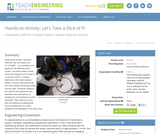Conditions of Use:
Rating

Working as a team, students discover that the value of pi (3.1415926...) is a constant and applies to all different sized circles. The team builds a basic robot and programs it to travel in a circular motion. A marker attached to the robot chassis draws a circle on the ground as the robot travels the programmed circular path. Students measure the circle's circumference and diameter and calculate pi by dividing the circumference by the diameter. They discover the pi and circumference relationship; the circumference of a circle divided by the diameter is the value of pi.

Subject:
Engineering
Mathematics
Material Type:
Activity/Lab
Provider:
TeachEngineering
Provider Set:
TeachEngineering
Author:
Carole Chen
Michael Hernandez
09/18/2014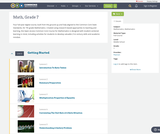Conditions of Use:
Remix and Share
Rating

Four full-year digital course, built from the ground up and fully-aligned to the Common Core State Standards, for 7th grade Mathematics. Created using research-based approaches to teaching and learning, the Open Access Common Core Course for Mathematics is designed with student-centered learning in mind, including activities for students to develop valuable 21st century skills and academic mindset.

Subject:
Mathematics
Material Type:
Full Course
Provider:
Pearson
10/06/2016Conditions of Use:
Remix and Share
Rating

Algebraic Reasoning

Type of Unit: Concept

Prior Knowledge

Students should be able to:

Add, subtract, multiply, and divide rational numbers.
Evaluate expressions for a value of a variable.
Use the distributive property to generate equivalent expressions including combining like terms.
Understand solving an equation or inequality as a process of answering a question: which values from a specified set, if any, make the equation or inequality true?
Write and solve equations of the form x+p=q and px=q for cases in which p, q, and x are non-negative rational numbers.
Understand and graph solutions to inequalities x<c or x>c.
Use equations, tables, and graphs to represent the relationship between two variables.
Relate fractions, decimals, and percents.
Solve percent problems included those involving percent of increase or percent of decrease.

Lesson Flow

This unit covers all of the Common Core State Standards for Expressions and Equations in Grade 7. Students extend what they learned in Grade 6 about evaluating expressions and using properties to write equivalent expressions. They write, evaluate, and simplify expressions that now contain both positive and negative rational numbers. They write algebraic expressions for problem situations and discuss how different equivalent expressions can be used to represent different ways of solving the same problem. They make connections between various forms of rational numbers. Students apply what they learned in Grade 6 about solving equations such as x+2=6 or 3x=12 to solving equations such as 3x+6=12 and 3(x−2)=12. Students solve these equations using formal algebraic methods. The numbers in these equations can now be rational numbers. They use estimation and mental math to estimate solutions. They learn how solving linear inequalities differs from solving linear equations and then they solve and graph linear inequalities such as −3x+4<12. Students use inequalities to solve real-world problems, solving the problem first by arithmetic and then by writing and solving an inequality. They see that the solution of the algebraic inequality may differ from the solution to the problem.

Subject:
Mathematics
Algebra
Material Type:
Unit of Study
Provider:
Pearson